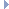# OpenGraph DrawingFramework

v. 2022.02 (Dogwood)

ogdf::MinSTCutBFS< TCost > Class Template Reference

Min-st-cut algorithm, that calculates the cut by doing a depth first search over the dual graph of of an st-planar input graph. More...

#include <ogdf/graphalg/MinSTCutBFS.h>Inheritance diagram for ogdf::MinSTCutBFS< TCost >:

## Public Member Functions

MinSTCutBFS ()

virtual bool call (const Graph &graph, const EdgeArray< TCost > &weight, node s, node t, List< edge > &edgeList, edge e_st=nullptr) override
The actual algorithm call. More...

virtual bool call (const Graph &graph, node s, node t, List< edge > &edgeList, edge e_st=nullptr) override
The actual algorithm call. More...Public Member Functions inherited from ogdf::MinSTCutModule< TCost >
MinSTCutModule ()
default constructor (empty) More...

virtual ~MinSTCutModule ()

virtual bool direction (edge e)
Returns the direction of e in the cut. More...

## Private Member Functions

bool call (const Graph &graph, const EdgeArray< TCost > *weight, node s, node t, List< edge > &edgeList, edge e_st)
This internal call uses a pointer to the weight instead of a reference. More...Protected Member Functions inherited from ogdf::MinSTCutModule< TCost >
bool preprocessingDual (const Graph &graph, GraphCopy &gc, CombinatorialEmbedding &CE, node source, node target, edge e_st)
This method preprocesses gc for minstcut calculations, by adding an st-edge if needed and embedding gc. More...Protected Attributes inherited from ogdf::MinSTCutModule< TCost >
EdgeArray< int > m_direction

GraphCopym_gc = nullptr

## Detailed Description

### template<typename TCost> class ogdf::MinSTCutBFS< TCost >

Min-st-cut algorithm, that calculates the cut by doing a depth first search over the dual graph of of an st-planar input graph.

Precondition
The input graph is st-planar.
Template Parameters
 TCost The type in which the weight of the edges is given.

Definition at line 54 of file MinSTCutBFS.h.

## ◆ MinSTCutBFS()

template<typename TCost >
 ogdf::MinSTCutBFS< TCost >::MinSTCutBFS ( )
inline

Definition at line 56 of file MinSTCutBFS.h.

## ◆ call() [1/3]

template<typename TCost >
 virtual bool ogdf::MinSTCutBFS< TCost >::call ( const Graph & graph, const EdgeArray< TCost > & weight, node s, node t, List< edge > & edgeList, edge e_st = nullptr )
inlineoverridevirtual

The actual algorithm call.

Parameters
 graph The graph on which the min-st-cut is to be calculated. weight Provides a weight for every edge. s The source node. t The target node. edgeList This list is filled with the edges which are part of the mincut. If the graph is st-planarly embedded, this list is correctly ordered along the cut. e_st An edge between s and t which is used to determine where the cut should start, nullptr elsewise.
Returns
Indicates success.

Implements ogdf::MinSTCutModule< TCost >.

Definition at line 69 of file MinSTCutBFS.h.

## ◆ call() [2/3]

template<typename TCost >
 bool ogdf::MinSTCutBFS< TCost >::call ( const Graph & graph, const EdgeArray< TCost > * weight, node s, node t, List< edge > & edgeList, edge e_st )
private

This internal call uses a pointer to the weight instead of a reference.

The actual algorithm call.

Parameters
 graph The graph on which the min-st-cut is to be calculated. weight Provides a weight for every edge. s The source node. t The target node. edgeList This list is filled with the edges which are part of the mincut. If the graph is st-planarly embedded, this list is correctly ordered along the cut. e_st An edge between s and t which is used to determine where the cut should start, nullptr elsewise.
Returns
Indicates success.

Definition at line 87 of file MinSTCutBFS.h.

## ◆ call() [3/3]

template<typename TCost >
 virtual bool ogdf::MinSTCutBFS< TCost >::call ( const Graph & graph, node s, node t, List< edge > & edgeList, edge e_st = nullptr )
inlineoverridevirtual

The actual algorithm call.

Parameters
 graph The graph on which the min-st-cut is to be calculated. s The source node. t The target node. edgeList This list is filled with the edges which are part of the mincut. If the graph is st-planarly embedded, this list is correctly ordered along the cut. e_st An edge between s and t which is used to determine where the cut should start, nullptr elsewise.
Returns
Indicates success.

Implements ogdf::MinSTCutModule< TCost >.

Definition at line 62 of file MinSTCutBFS.h.

The documentation for this class was generated from the following file: